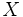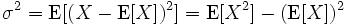# Probability and Statistics

This is the current revision of Probability and Statistics as edited by at 17:52, 13 July 2016. This URL is a permanent link to this version of this page.

(diff) ← Older revision | Latest revision (diff) | Newer revision → (diff)This article/section deals with mathematical concepts appropriate for late high school or early college.

Variance is a measure in statistics of the dispersion of a set of values (represented as). It is defined aswhere the expected value of X is E(X).

The formula for variance must not be confused with the formulawhich is the formula for a point estimate of the true variance from a sample of size n. As such this estimator itself has a variance which, as the formula indicates, decreases as the sample size increases.